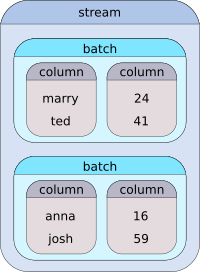HiPipe  0.7.0 C++17 data pipeline with Python bindings.
Introduction

# Preface

HiPipe is a C++ library for efficient data processing. Its main purpose is to simplify and accelerate data preparation for deep learning models, but it is generic enough to be used in many other areas.

HiPipe lets the programmer build intuitive data streams that transform, combine and filter the data that pass through. Those streams are compiled, batched, and asynchronous, therefore maximizing the utilization of the provided hardware.

The complete source code can be downloaded from our official GitHub repository.

This project is under heavy development. The API is continuously changing without regard to backward compatibility.

# Quick Start

Before proceeding, please install HiPipe by following the installation guide.

HiPipe provides many smaller utilities, such as loading CSV files to dataframes, base64 decoders, data splitting and much more. However, probably the most useful funcionality of the library is data stream processing.

Before we dive into details, we recommend to get familiar with the concept of C++ ranges and Range-v3 library by Eric Niebler. Ranges will soon become a fundamental part of C++ standard and Range-v3 library is an experimental implementation of the proposal. To sum up the idea, a range can be thought of as a pair of begin/end iterators with potentially infinite distance.

Now, a stream in the context of HiPipe is a range of batches, where each batch contains one or more columns. To demonstrate this concept on an example, let us assume that we have a table of four users and each user has properties: `login` and `age`:

marry 24
ted 41
anna 16
josh 59

A stream made of such data with batch size of two may look as following:Of course, the stream may as well be loaded from a file or a database and may even be infinite. HiPipe provides many tools to manipulate such streams using the pipe "|" operator. The following example demonstrates the main idea of stream pipelining (see the full example in example.cpp):

namespace hps = hipipe::stream;
using hps::from; using hps::to; using hps::by; using hps::dim;
HIPIPE_DEFINE_COLUMN(login, std::string) // helper macro to define a column of strings
std::vector<std::string> logins = {"marry", "ted", "anna", "josh"};
std::vector<int> ages = { 24, 41, 16, 59};
// create a batched stream out of the raw data
// make everyone older by one year
| hps::transform(from<age>, to<age>, [](int a) { return a + 1; })
// increase each letter in the logins by one (i.e., a->b, e->f ...)
| hps::transform(from<login>, to<login>, [](char c) { return c + 1; }, dim<2>)
// increase the ages by the length of the login
| hps::transform(from<login, age>, to<age>, [](std::string l, int a) {
return a + l.length();
})
// probabilistically rename 50% of the people to "buzz"
return "buzz";
})
// drop the login column from the stream
// introduce the login column back to the stream
| hps::transform(from<age>, to<login>, [](int a) {
return "person_" + std::to_string(a) + "_years_old";
})
// filter only people older than 30 years
| hps::filter(from<login, age>, by<age>, [](int a) { return a > 30; })
// asynchronously buffer the stream during iteration
// extract the ages from the stream to std::vector
ages = hps::unpack(stream, from<age>);
assert((ages == std::vector<int>{45, 64}));

# Multidimensional data

In HiPipe, when we talk about multidimensional data, we are talking about nested ranges. For instance, `std::vector<int>` is a one dimensional vector and `std::vector<std::vector<int>>` is a two dimensional vector.

Most of the stream modifiers have a special parameter called `dim<N>`, that denotes in which dimension should the modifier operate. For instance, consider the transform() from our previous example:

...
// This transformation is applied in dimension 1 (default), so in the case of the
// login column, the transformation function expects std::string.
// This transformation is applied in dimension 2, which for std::string means
// that it is applied on each letter.
| hps::transform(from<login>, to<login>, [](char c) { return c + 1; }, dim<2>)
// And this transformation is applied in dimension 0, which denotes that the function
// expects the whole batch.
}, dim<0>)

In HiPipe, there are no constraints on the shape of the columns. Batch sizes can differ batch to batch, as well as the sizes of the data across a single dimension. For instance, it is possible to have a batch of variably long sequences (e.g., a batch of video frames from videos of different length) and stream modifiers, such as transform() and filter(), will handle it in any dimension you choose. To define a column containing multidimensional data, the most simple solution is to wrap the data in `std::vector` (but any reasonable container will suffice):

HIPIPE_DEFINE_COLUMN(stock_price_evolution, std::vector<double>)

# Python

HiPipe provides a convenient functions to convert and stream data to Python. This part of the documentation is yet to be prepared. Meanwhile, please refer to the Python binding API documentation.

hipipe::utility::to_string
std::string to_string(const T &value)
Convert the given type to std::string.
Definition: string.hpp:90
hipipe::stream::filter
auto filter(from_t< FromColumns... > f, by_t< ByColumns... > b, Fun fun, dim_t< Dim > d=dim_t< 1 >{})
Filter stream data.
Definition: filter.hpp:154
hipipe::stream::buffer
rgv::view< buffer_fn > buffer
Asynchronously buffers the given range.
Definition: buffer.hpp:195
HIPIPE_DEFINE_COLUMN
#define HIPIPE_DEFINE_COLUMN(column_name_, example_type_)
Macro for fast column definition.
Definition: column_t.hpp:250
hipipe::stream::unpack
auto unpack(Rng &&rng, from_t< FromColumns... > f, dim_t< Dim > d=dim_t< 1 >{})
Unpack a stream into a tuple of ranges.
Definition: unpack.hpp:118
hipipe::stream::transform
auto transform(from_t< FromColumns... > f, to_t< ToColumns... > t, Fun fun, dim_t< Dim > d=dim_t< 1 >{})
Transform a subset of hipipe columns to a different subset of hipipe columns.
Definition: transform.hpp:218